# Metallurgical Engineering - MT 2013 GATE Paper (Practice Test)

## 65 Questions MCQ Test GATE Past Year Papers for Practice (All Branches) | Metallurgical Engineering - MT 2013 GATE Paper (Practice Test)

Description
Attempt Metallurgical Engineering - MT 2013 GATE Paper (Practice Test) | 65 questions in 180 minutes | Mock test for GATE preparation | Free important questions MCQ to study GATE Past Year Papers for Practice (All Branches) for GATE Exam | Download free PDF with solutions
QUESTION: 1

Solution:
QUESTION: 2

Solution:
QUESTION: 3

### Which of the following options is the closest in meaning to the word given below: Primeval

Solution:
QUESTION: 4

Friendship, no matter how _________it is, has its limitations.

Solution:
QUESTION: 5

Select the pair that best expresses a relationship similar to that expressed in the pair:
Medicine: Health

Solution:
QUESTION: 6

Q. 6 to Q. 10 carry two marks each.

X and Y are two positive real numbers such that 2X + Y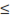6 and X + 2Y8. For which of the following values of (X,Y),  the function f(X,Y)=  3X + 6Y will give maximum value?

Solution:
QUESTION: 7

If |4X - 7| = 5 then the values of 2|X| - |-X| is:

Solution:
QUESTION: 8

Following table provides figures (in rupees) on annual expenditure of a firm for two years - 2010 and 2011.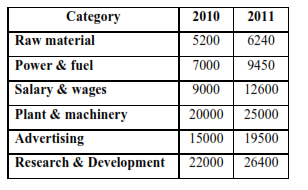In 2011, which of the following two categories have registered increase by same percentage?

Solution:
QUESTION: 9

A firm is selling its product at Rs. 60 per unit. The total cost of production is Rs. 100 and firm is earning total profit of Rs. 500. Later, the total cost increased by 30%. By what percentage the price should be increased to maintained the same profit level.

Solution:
QUESTION: 10

Abhishek is elder to Savar.
Savar is younger to Anshul.

Which of the given conclusions is logically valid and is inferred from the above statements?

Solution:
QUESTION: 11

USEFUL DATA
Universal gas constant, R: 8.314 J/mol/K
Acceleration due to gravity, g: 9.81 m/s2
Bohr magneton, μB: 9.273 x 10-24 Am2

Q. 11 – Q. 35 carry one mark each.

Degree and order of the differential equation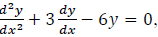respectively, are

Solution:
QUESTION: 12

As the concentration of point defects in a crystal increases, its configurational entropy

Solution:
QUESTION: 13

In a binary system A-B, εAA, εBB and εAB correspond to A-A, B-B and A-B bond energies respectively. The miscibility gap will occur if

Solution:
QUESTION: 14

Critical value of the Gibbs energy of nucleation at equilibrium temperature is

Solution:
QUESTION: 15

With respect to the matrix of Al-Cu alloys, G-P zones are

Solution:
QUESTION: 16

Which one of the following techniques does NOT require quenching to obtain final case hardness?

Solution:
QUESTION: 17

Which one of the following elements is an austenite stabilizer?

Solution:
QUESTION: 18

A 0.2 wt.% plain carbon steel sheet is heated and equilibrated in the inter-critical region followed by instant water quenching. The microstructure of the quenched steel sheet consists of

Solution:
QUESTION: 19

As compared to the engineering stress-engineering strain curve, the true stress-true strain curve for a given material

Solution:
QUESTION: 20

Which one of the following does NOT improve fatigue life of a steel component?

Solution:
QUESTION: 21

When two phases α and β in an alloy are in thermodynamic equilibrium, then

Solution:
QUESTION: 22

Isothermal compressibility of a material is given by

Solution:
QUESTION: 23

In the Ellingham diagram for oxides, C-CO line cuts the M-MO line at temperature T1 and the M′-M′O line at a higher temperature T2. At a temperature greater than T1 and less than T2, carbon can reduce

Solution:
QUESTION: 24

Which one of the following can give information about the corrosion rate?

Solution:
QUESTION: 25

In a roasting process, the set of conditions that favour sulphate formation from metal sulphide concentrates are

P. high temperature
Q. high partial pressure of oxygen
R. use of excess air
S. high partial pressure of SO3

Solution:
QUESTION: 26

High top pressure in a blast furnace operation

Solution:
QUESTION: 27

In L-D steelmaking, the final slag can be best described as

Solution:
QUESTION: 28

The permeability of burden in an ironmaking blast furnace can be improved by using

Solution:
QUESTION: 29

For a good quality brazing, the molten filler alloy should have

Solution:
QUESTION: 30

Risers are NOT required for casting

Solution:
*Answer can only contain numeric values
QUESTION: 31

For scalar fields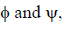the value of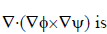______

Solution:
*Answer can only contain numeric values
QUESTION: 32

The atomic packing fraction of diamond cubic structure is __________

Solution:
*Answer can only contain numeric values
QUESTION: 33

The total number of possible heat transfer mode(s) is __________

Solution:
*Answer can only contain numeric values
QUESTION: 34

If σ and ε are true stress and true strain, respectively, the maximum true uniform strain that can be
imparted to a material obeying σ = 1050ε0.25 is _________

Solution:
*Answer can only contain numeric values
QUESTION: 35

Arc welding is done using current, voltage and welding speed of 200 A, 20 V and 0.01 m/s, respectively. The heat input in kJ per unit length is _________

Solution:
QUESTION: 36

Q. 36 to Q. 65 carry two marks each.

Which one of the following series is divergent?

Solution:
QUESTION: 37

Taylor series expansion of the function f(x)= x/1+x around x = 0 will be

Solution:
QUESTION: 38

Which one of the following attributes is NOT correct for the matrix?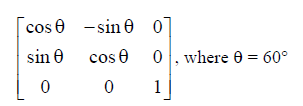Solution:
QUESTION: 39

A unit cell of an element has maximum linear density along the  direction. The packing density of its (100) plane is

Solution:
QUESTION: 40

For an FCC metal, the ratio of interplanar spacing obtained from the first two peaks of the X-ray diffraction pattern is

Solution:
QUESTION: 41

There are 150 gearwheels in a box, out of which 112 are within the required tolerance, 21 are below and rest are above the required tolerance. If the selection is done without replacement, the combined probability of randomly selecting a gearwheel below the tolerance and then a second one above the tolerance is

Solution:
QUESTION: 42

Match the metal in Group I with its corresponding ore in Group II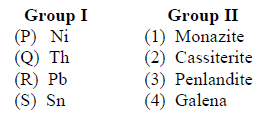Solution:
QUESTION: 43

The yield strength of a polycrystalline metal increases from 100 MPa to 145 MPa on decreasing the grain size from 64 μm to 25 μm. The yield strength of this metal (in MPa) having a grain size of 36 μm is

Solution:
QUESTION: 44

In a brittle material, the maximum internal crack length is 8 μm. If Young’s modulus is 400 GPa and surface energy is 3.14 J/m2, the estimated theoretical fracture strength (in MPa) is

Solution:
QUESTION: 45

Saturation magnetization of an FCC metal with lattice parameter 0.2 nm is 600 kA/m. The net magnetic moment per atom is given by (in Bohr magneton)

Solution:
QUESTION: 46

A 480 mm thick slab is hot-rolled using a roll of 720 mm diameter. For a coefficient of friction of 0.5, the maximum possible reduction (in mm) is

Solution:
QUESTION: 47

Match the defects listed in Group I with the corresponding manufacturing process listed in Group II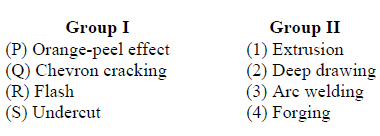Solution:
QUESTION: 48

Match the powder production technique given in Group I with the corresponding shape listed in Group II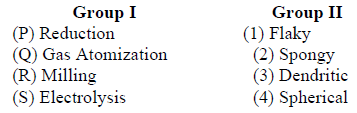Solution:
QUESTION: 49

Match the suitability of non-destructive testing method in Group I for the detection of defects listed in Group II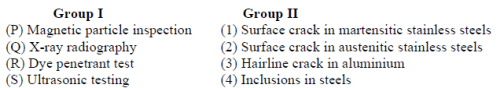Solution:
QUESTION: 50

For the following electrochemical reaction Sn + 2H+ = Sn2+ + H2, if the solution has Sn2concentration 10-2 M and pH 5 at 298 K, which of the following is true? Given: standard reduction potential for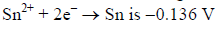versus SHE; pH2 = 1 atm

Solution:
QUESTION: 51

Match the unit operation in Group I with its corresponding principle in Group II: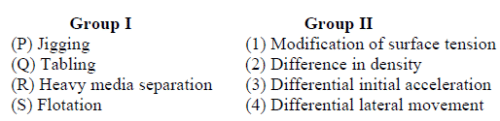Solution:
QUESTION: 52

Determine the correctness or otherwise of the following Assertion (a) and Reason (r).
Assertion: For the extraction of metal values from their sulphide concentrates by hydrometallurgical route, leaching with oxygen under high pressure is used.
Reason: Presence of oxygen under high pressure causes roasting of sulphides, which helps in leaching of the values.

Solution:
*Answer can only contain numeric values
QUESTION: 53

The aperture size (in μm) of a 200 mesh sieve having a wire diameter of 53 μm is __________

Solution:
*Answer can only contain numeric values
QUESTION: 54

From a 2 m × 1.2 m sheet, squares are cut out from each of the four corners as shown in the figure and then the sides are bent to form an open box. The maximum possible volume (in m3) of the box is __________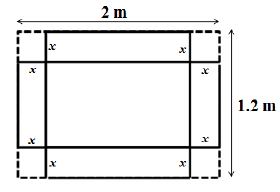Solution:
*Answer can only contain numeric values
QUESTION: 55

Applying the secant method, the first approximation to the root of f(x)=  1 + ln x+ x/2, starting with function values at x = 0.3 and x = 0.4, is_______

Solution:
*Answer can only contain numeric values
QUESTION: 56

The critical internal crack length (in mm) in a steel having KIc of 45 MPa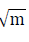to support a Mode-I stress of 400 MPa is __________

Solution:
*Answer can only contain numeric values
QUESTION: 57

Ladle deoxidation of liquid steel is done at 1600°C by adding ferro-aluminium. By assuming Stokes law behaviour, time (in s) required for alumina particles of 50 μm diameter to float to the surface from a depth of 2 m would be__________

[Given: density of steel = 7000 kg/m3, density of alumina = 3650 kg/m3, viscosity of steel = 6*10-3 kg/m/s]

Solution:
QUESTION: 58

Common Data Questions

Common Data for Questions 58 and 59:

A steel specimen containing 0.2 wt.% C is carburized in an atmosphere that maintains a carbon content of 1.2 wt.% C at the surface of the specimen.

Given:
For carbon diffusion in austenite: D0=2.0×10-5 m2/s
Activation energy for diffusion, Q=142 kJ/mol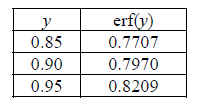What is the depth (in μm) from the surface of the specimen at which a composition of 0.4 wt.% C is obtained after carburizing at 870°C for 10 h?

Solution:
QUESTION: 59

A steel specimen containing 0.2 wt.% C is carburized in an atmosphere that maintains a carbon content of 1.2 wt.% C at the surface of the specimen.

Given:
For carbon diffusion in austenite: D0=2.0×10-5 m2/s
Activation energy for diffusion, Q=142 kJ/molHow long (in h) will it take to double the depth at which 0.4 wt.% C is reached?

Solution:
QUESTION: 60

Common Data for Questions 60 and 61:

Integral enthalpy of mixing (in J/mol) of liquid (Cu, Zn) solution can be approximated by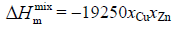The corresponding partial molar enthalpy of mixing (in J/mol) for Cu is

Solution:
QUESTION: 61

Integral enthalpy of mixing (in J/mol) of liquid (Cu, Zn) solution can be approximated byAssuming regular solution behaviour, the solution parameter (in J/mol) is

Solution:
QUESTION: 62

The density and associated crystallinity for two polypropylene samples are as follows: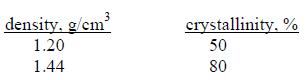Density of totally amorphous polypropylene is

Solution:
QUESTION: 63

The density and associated crystallinity for two polypropylene samples are as follows:The percent crystallinity of polypropylene sample having a density of 1.3 g/cm3 is

Solution:
QUESTION: 64

An edge dislocation is present in α-Fe. Atomic diameter of iron atom is 0.25 nm and its shear modulus is 70 GPa.

Modulus of the Burgers vector (in nm) is

Solution:
QUESTION: 65

An edge dislocation is present in α-Fe. Atomic diameter of iron atom is 0.25 nm and its shear modulus is 70 GPa.

Energy (in J/m) of the dislocation is

Solution:Use Code STAYHOME200 and get INR 200 additional OFF Use Coupon Code